# Project Overview

My final project is an extended version of the software rasterizer our class implemented for a lab assignment. The initial rasterizer was only required to support simple diffuse shading and Gaussian blur. For my project, I added support for screen-space ambient occlusion, alpha blending, and texture mapping. Each of these extensions is described in more detail below.

# Features

## Screen-Space Ambient Occlusion

This effect is an approximation of ambient occlusion, which reduces ambient lighting for recessed portions of a model. Ambient occlusion is illustrated in the following image.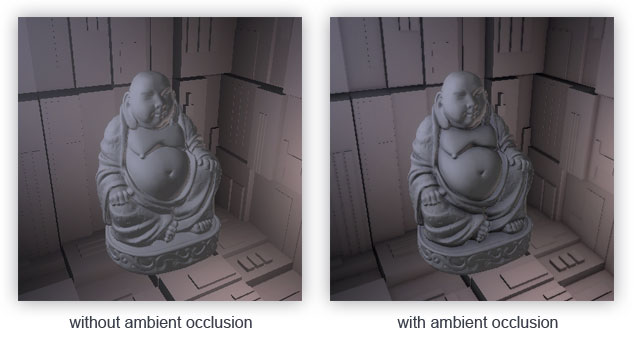Unlike standard ambient occlusion, screen-space ambient occlusion operates entirely in image space, and is fast enough for real-time applications [citation?]. Screen-space ambient occlusion can be summarized as follows:

``` For each pixel in the frame     Sample a set of nearby locations in the Z-buffer    For each sampled location        If it is in front of the current pixel, increase the current pixel's occlusion factor    Strength of occlusion effect is proportional to occlusion factor```

My rasterizer's implementation of screen-space ambient occlusion samples all Z-buffer values in an 11x11 box around each pixel in the frame. Since the camera is at the origin looking down the negative Z-axis, points with greater Z values than the current pixel contribute to the pixel's occlusion factor. Each sample location's contribution is scaled by its distance in screen-space from the current pixel, so that more distant points have less influence on the occlusion effect. The individual sample locations' contributions are then summed to obtain an initial occlusion factor for the pixel.

In order to prevent contributions from points with Z values much greater or much less than the current pixel's Z value (such as background pixels), my rasterizer's SSAO implementation includes a "range check" as described in . A sample location only contributes to the current pixel's occlusion factor if the difference between their Z values is less than a specified threshold.

It is important to note that, at this stage, sample locations equidistant from the current pixel contribute equally to the pixel's occlusion factor, regardless of their "height" compared to the pixel's Z position. Since deeply recessed points should be more occluded than points in shallower depressions on the mesh, my rasterizer scales each pixel's occlusion factor by the average depth difference between the pixel and its neighbors.

Finally, the occlusion factor is normalized to fall between 0 and 1, and is then inverted - that is, if the occlusion factor is F, then F becomes 1 - F. This value is then used to scale the corresponding pixel's RGB values, such that the pixel's final color is its original color multiplied by the occlusion factor.

The following images demonstrate the screen-space ambient occlusion effect. The image on the left was generated without SSAO, while the image on the right was generated with SSAO enabled.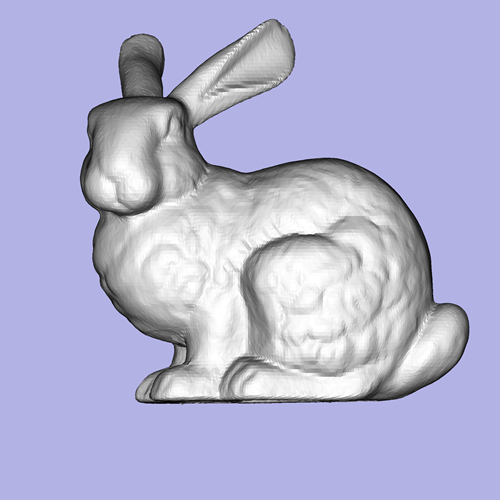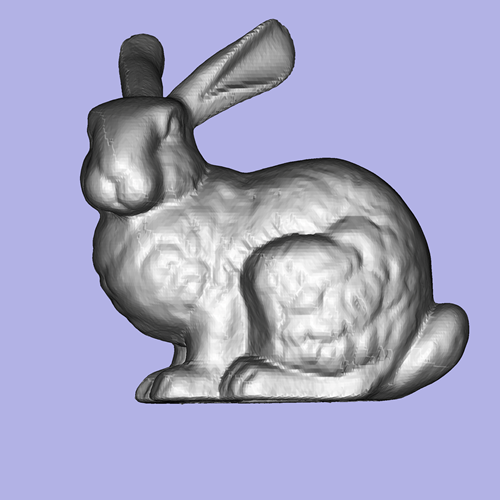## Alpha Blending

For this project, I used alpha blending to implement simple transparency effects. Each object in the scene is given an alpha value, which ranges from 0 (fully transparent) to 1 (fully opaque). My rasterizer uses the A-buffer technique discussed in . An A-buffer stores a list of fragments for each pixel location on the screen. As objects are drawn, their colors are added to the A-buffer at the appropriate screen coordinates. When all objects have been drawn, the A-buffer's lists are sorted by Z value, and each list's colors are blended in order (either back-to-front or front-to-back). The following image illustrates the A-buffer data structure.My rasterizer implements alpha blending by rasterizing each object in the scene separately. After an object has been rasterized, the frame buffer's contents (excluding background points) are added to the A-buffer, which is implemented as a 2D array of C++ STL priority_queues. Each pixel color added to the A-buffer also includes the model's alpha value. The frame buffer is then cleared before other objects are rasterized. Finally, when all objects have been rasterized, each pixel location's corresponding list of colors in the A-buffer is blended using the following recursive formula, where color_n is the blended color for layer n, pixel_n is the fragment (RGB) at layer n, and alpha_n is the alpha value for the fragment at layer n:

`color_n = alpha_n * pixel_n + (1 - alpha_n) * color_n-1`

The resulting colors are then written back to the frame buffer to produce the final image. Below is an example image from my rasterizer that demonstrates alpha blending.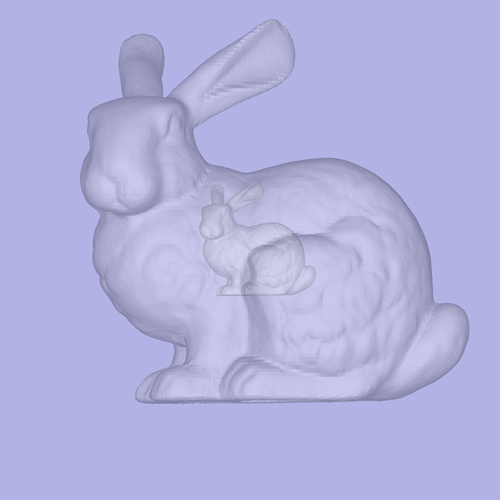## Texture Mapping

My rasterizer supports basic texture mapping using spherical parameterization. In brief, this technique treats points on the mesh being rendered as points on a sphere, and calculates U and V (texture coordinates) accordingly. Texture mapping is performed during the rasterization process. For each pixel to be drawn, the rasterizer converts the pixel's screen coordinates to world coordinates in the range [-1..1], and then uses the world X and Y coordinates to derive the corresponding Z value for a point at the given X and Y on a unit sphere. These world coordinates are used to calculate U and V as follows:

`v = acos(worldY) / PI;`

```if (sphereZ >= 0)    u = acos(worldX / sin(PI * v)) / (2*PI);else    u = (PI + acos(worldX / sin(PI * v)) / (2*PI);```

Pixel coordinates for the desired color in the texture are calculated as (u*textureWidth, v*textureHeight). The color at this location in the texture is then used to scale the shade of gray calculated for the pixel via linear interpolation, producing the final color for the pixel.

This image is an example texture-mapped model generated by my rasterizer.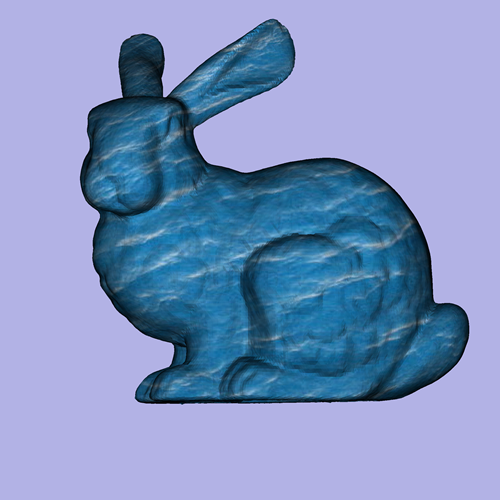# All Extensions Combined

This image is a composite scene generated with screen-space ambient occlusion, alpha blending, and texture mapping enabled simultaneously.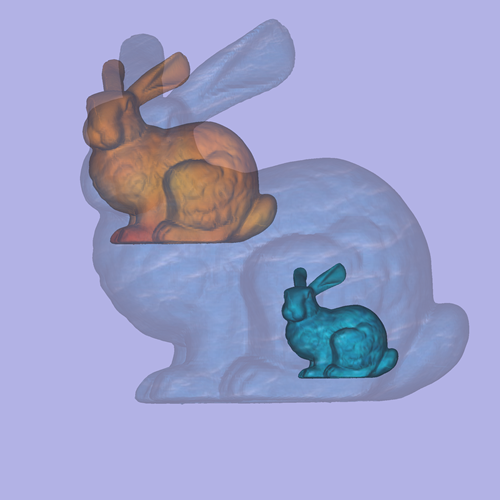# References

 M. Maule, J. L.D. Comba, R. P. Torchelsen, R. Bastos, "A survey of raster-based transparency techniques," Computers & Graphics, vol. 35, issue 6, December 2011, pp. 1023-1034. [Online]. Available: http://www.sciencedirect.com/science/article/pii/S009784931100135X